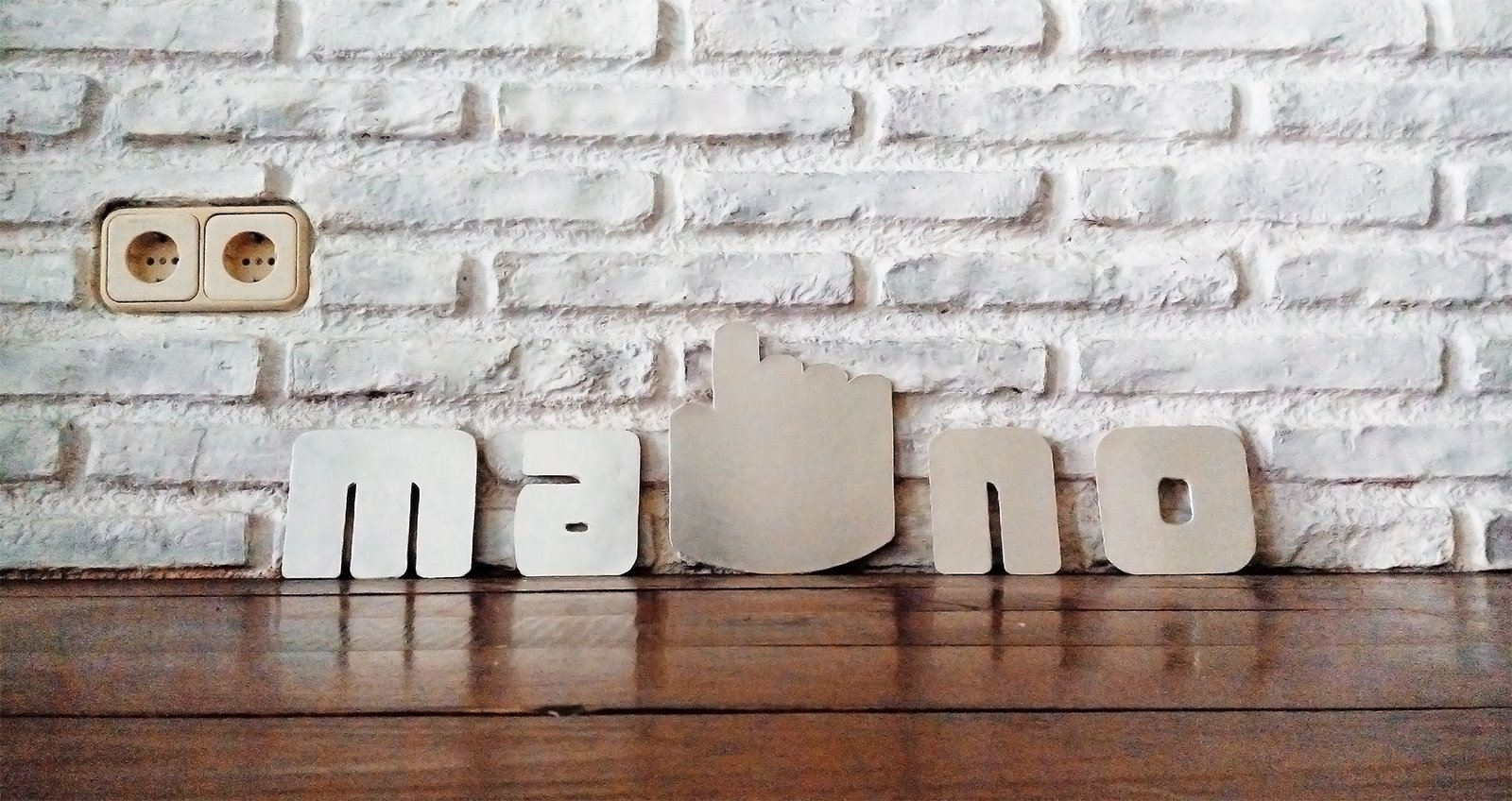# Six Useful PHP code snippetsby Janeth Kent Date: 11-04-2013

Here’s a mini list of  useful PHP code snippets that we find using on a weekly basis. You could use these as is or expand them as part of other applications or add them to a php class.

Useful if you have a server in a different timezone, like us... You can fix funny things happen when you add the time to a MySQL database, by adding or subtracting hours as in the example below.

\$now = date('Y-m-d-G');
\$now = strftime("%Y-%m-%d-%H", strtotime("\$now -8 hours"));

### Convert HEX value to RBG

function hextorgb(\$hexvalue){

 if(\$hexvalue == '#') {
 \$hexvalue = substr( \$hexvalue, 1);
 }
 if(strlen( \$hexvalue ) == 6){
 list(\$r, \$g, \$b) = array(\$hexvalue .\$hexvalue, \$hexvalue . \$hexvalue, \$hexvalue .\$hexvalue);
 }elseif (strlen(\$hexvalue) == 3) {
 list(\$r,\$g,\$b) = array(\$hexvalue .\$hexvalue, \$hexvalue . \$hexvalue, \$hexvalue .\$hexvalue);
 }else{
 return false;
 }
 \$r = hexdec(\$r);
 \$g = hexdec(\$g);
 \$b = hexdec(\$b);
 return array('R' => \$r, 'G' => \$g, 'B' => \$b);
 }
 \$rgb = hextorgb('#fff000');
print_r(\$rgb);

### Display a users Gravatar image

 \$reg_exUrl = "/(http|https|ftp|ftps)\:\/\/[a-zA-Z0-9\-\.]+\.[a-zA-Z]{2,3}(\/\S*)?/";
 // Check if there is a url in the string
 if (preg_match_all(\$reg_exUrl, \$text, \$url)) {
 foreach(\$url as \$v){
 \$position = strpos(\$text,' '.\$v)+1;
 \$text = substr_replace(\$text,'',\$position, strlen(\$v));
 \$text = substr_replace(\$text,''.\$v.'',\$position ,0);
 }
 return \$text;
 }
 else {
 // if no urls in the text just return the text
 return \$text;
 }
 }
 \$string = 'This is a string of text and we have a link: http://ma-no.org we also have another link http://google.com';

### Parse JSON in PHP

\$json='{"id":0,"name":"Silvia","surname":"Salvietta","Website":"http://ma-no.org"} ';

 \$array=json_decode(\$json);
 // print  the array
 print_r(\$array);
echo \$array->name;

by Janeth Kent Date: 11-04-2013 hits : 2793#### Janeth Kent

Licenciada en Bellas Artes y programadora por pasión. Cuando tengo un rato retoco fotos, edito vídeos y diseño cosas. El resto del tiempo escribo en MA-NO WEB DESIGN AND DEVELOPMENT.